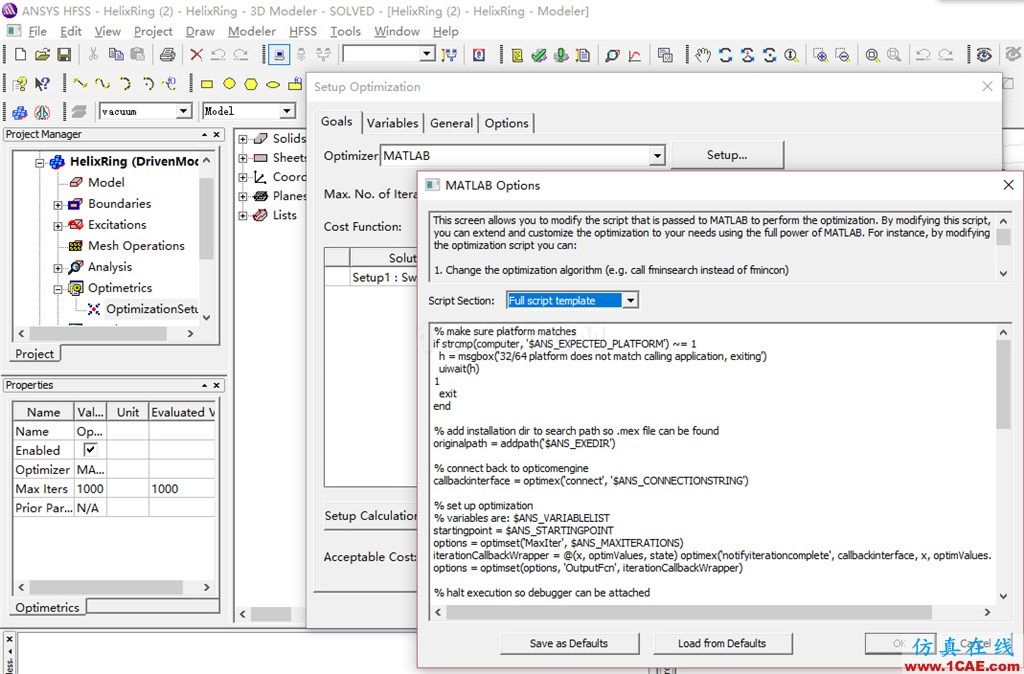# 使用HFSS优化工具中的MATLAB选项的一个问题

2017-04-05  by:CAE仿真在线  来源:新浪博文

[error] Engine : Initial connect from MATLAB never received, aborting.

% make sure platform matches

if strcmp(computer, 'PCWIN64') ~= 1

h = msgbox('32/64 platform does not match calling application, exiting')

uiwait(h)

exit

end## MATLAB optimizer

The MATLAB optimizer option lets you pass a script to MATLAB to perform the optimization. When the optimization is analyzed, MATLAB is launched and a script is passed in to MATLAB to perform the optimization. During the optimization, MATLAB will call back into our application to perform the solve and compute the cost. The cost will be reported back to MATLAB, and MATLAB's optimization will determine the next step in the optimization.

• 客服在线请直接联系我们的客服，您也可以通过下面的方式进行在线报名，我们会及时给您回复电话，谢谢！